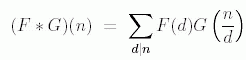ENG  RUS Timus Online JudgeOnline Judge
Problems
Authors
Online contests
Site news
Webboard
Problem set
Submit solution
Judge status
Guide
Register
Authors ranklist
Current contest
Scheduled contests
Past contests
Rules

## 1554. Multiplicative Functions

Time limit: 2.0 second
Memory limit: 64 MB
In number theory, a multiplicative function is an arithmetic function F(n) of the positive integer n with property that F(1) = 1 and whenever a and b are coprime (gcd(a, b) = 1), then F(ab) = F(a)F(b).
The function E(n) defined by E(n) = 1 if n = 1 and = 0 if n > 1, is sometimes called multiplication unit for Dirichlet convolution or simply the unit function. If F and G are two multiplicative functions, one defines a new multiplicative function F `*` G, the Dirichlet convolution of F and G, bywhere the sum extends over all positive divisors d of n. With this operation, the set of all multiplicative functions turns into an abelian group; the identity element is E.
In this task you have to find the inverse of a multiplicative function. To cope with overflow problem, we define arithmetic functions as: F: N —> Z2007 where N is the set of positive integers, and Z2007 is a residue ring (ring of integers 0–2006, where arithmetic operations + and × are performed modulo 2007). Function G is called the inverse of function F if and only if F `*` G = G `*` F = E.
You are given the first N values of function F, you need to find the first N values of the inverse function G.

### Input

In the first line of the input one number N is written (1 ≤ N ≤ 104). In the second line values F(1), F(2), F(3), …, F(N) are listed. Numbers are separated by spaces. (Each value is nonnegative and doesn't exceed 2006.)

### Output

In the first line of the output print first N values of inverse function G, separated by spaces: G(1), G(2), …, G(N).

### Sample

inputoutput
```16
1 1 1 1 1 1 1 1 1 1 1 1 1 1 1 1
```
```1 2006 2006 0 2006 1 2006 0 0 1 2006 0 2006 1 1 0
```
Problem Source: Novosibirsk SU Contest. Petrozavodsk training camp, September 2007